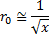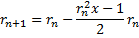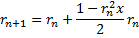# Inverse Square Root

## By Alexander Yee

(Last updated: February 4, 2019)

Back To:

y-cruncher uses Newton's Method iteration for performing the Inverse Square Root.

This operation is needed for the following constants:

• Square Root of x
• Golden Ratio
• Pi - Chudnovsky/Ramanujan

Algorithm

Find:Iterate:Since this is a 1st order iteration, the number of correct digits doubles with each iteration.

There are several ways to arrange the formula. This particular arrangement was chosen because rn2 x - 1 has destructive cancellation of roughly half the digits. This cancellation reduces the size of the final multiply by rn.

Implementation

In y-cruncher, the initial "guess" is obtained by simply calling std::sqrt() in <cmath> which gives 53 bits.

At each stage, y-cruncher's implementation will choose either of the following iterations:Which it chooses depends on whether rn turns out to be an under-estimate or an over-estimate of the correct answer. If it is an under-estimate, it uses the second formula to avoid negative numbers. Over-estimates are faster because subtraction by 1 is free whereas subtracting from 1 requires inversion of all the bits.

Therefore, y-cruncher's implementation will purturb the result of each iteration slightly to increase the chances that it will be an overestimate for the next iteration.

Lastly, there are two multiplications by rn.

• rn2
• rn * (...)

Since multiplies are the same size, y-cruncher reuses the forward FFT for rn.

Complexity Analysis

Complexity (Asymptotic):

Inverse Square Root: O( n log(n) )

The complexity of each iteration is O( M(n) ). Since Newton's Method is a self-correcting algorithm, full precision is not needed thoughout the iteration. Therefore the sum of all the iterations is a geometric sequence resulting in the same complexity: O( M(n) ).

If we assume that multiplication is M(n) = n log(n), then the entire operation is O( n log(n) ).

Complexity (Practical):

Memory: 1.33 M(n)

Swap: 1.50 M(n)

Each iteration contains:

• 1 Half-precision square
• 1 Half-precision multiply
• 1 Divide-by-two (negligible)

One forward FFT is reused for a total of 2 forward and 2 inverse FFTs.

For memory computations, we can assume that forward and inverse FFTs are of equal cost. Therefore a multiplication is equal to 3 FFTs.

For swap computations, the inverse FFT is about 2x the cost of a forward FFT. Therefore a multiplication is approximately 4 forward FFTs.

 Operation Size Quantity Total Cost Total Cost (Memory) Total Cost (Swap) Forward FFT 0.5 p 2 2 * F(0.5 p) 0.33 * M(p) 0.25 * M(p) Inverse FFT 0.5 p 2 2 * I(0.5 p) 0.33 * M(p) 0.50 * M(p) Add/Subtract 1.0 p 1 - - - Divide by 2 1.0 p 1 - - - Total 0.66 * M(p) 0.75 * M(p)

Summing these up through a binary geometric sequence gives exactly twice these numbers.